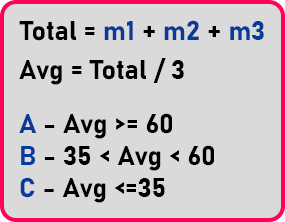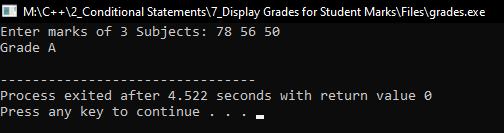# Display Grades for Student Marks in C++

## Display Grades for Student Marks in C++:

In this article, I am going to discuss How to Display Grades for Student Marks in C++ with Examples. Please read our previous article where we discussed How to Find Nature of Quadratic Roots in C++ with Examples.

##### Display Grades for Student Marks in C++

In this article, we will see another program where we will use ‘nested if’ conditions for showing the grades based on the student marks.Here are marks of 3 different subjects ‘m1’, ‘m2’ and ‘m3’. We will take the input of these 3 marks then find the total and average of these marks. After that based on the average marks, we will display grades for the students.

So, if average marks are greater than or equal to 60, we will print Grade ‘A’. If average marks are between 35 to less than 60 but greater than 35, we will print Grade ‘B’. And if the average marks are less than or equal to 35, then we will print Grade ‘C’.

So, we have just taken three grades. If you want you can increase grades as per your requirement. We will not display total marks and average marks; we will just display grades. Let us code this problem.

##### Display Grades for Student Marks Program in C++:
```#include <iostream>
using namespace std;

int main()
{
int m1, m2, m3, total;
float Avg;

cout << "Enter marks of 3 Subjects: ";
cin >> m1 >> m2 >> m3;

total = m1 + m2 + m3;
Avg = total / 3.0;

if (Avg >= 60)
cout << "Grade A" << endl;
else if (Avg > 35 && Avg < 60)
cout << "Grade B" << endl;
else
cout << "Grade C" << endl;

return 0;
}
```
###### Output:In the next article, I am going to discuss Else If Ladder in C++ with Examples. Here, in this article, I try to explain How to Display Grades for Student Marks in C++ with Examples and I hope you enjoy this How to Display Grades for Student Marks in C++ with Examples article.